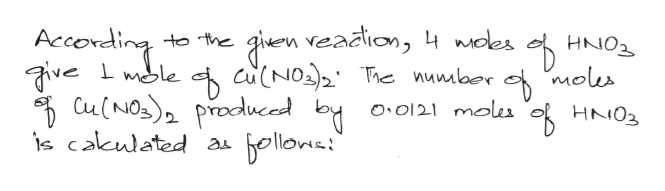# Using 0.0121 moles of HNO3 and the moles of Cu(NO3)2 produced from:1.) Cu(s) + 4HNO3(aq) --> Cu(NO3)2(aq) + 2NO2(g) + 2H2O(l)Calculate the total mass of sodium carbonate needed for: 2.) 2HNO3(aq) + Na2CO3(s) --> H2O(l) + CO2(g) + 2NaNO3(aq)3.) Cu(NO3)2(aq) + Na2CO3(s) --> CuCO3(s) + 2NaNO3(aq)Is 3.914 g of sodium carnbonate enough for the experiment?

Question

Using 0.0121 moles of HNO3 and the moles of Cu(NO3)2 produced from:

1.) Cu(s) + 4HNO3(aq) --> Cu(NO3)2(aq) + 2NO2(g) + 2H2O(l)

Calculate the total mass of sodium carbonate needed for:

2.) 2HNO3(aq) + Na2CO3(s) --> H2O(l) + CO2(g) + 2NaNO3(aq)

3.) Cu(NO3)2(aq) + Na2CO3(s) --> CuCO3(s) + 2NaNO3(aq)

Is 3.914 g of sodium carnbonate enough for the experiment?

check_circleExpert Solution
Step 1

We are authorized to answer one question at a time, since you have not mentioned which part you are looking for, so we are answering the first one, please repost your question separately for the remaining questions.

Step 2
Step 3help_outlineImage Transcriptionclosegern veadtion, 4waes Cu(NO2The nunlbor Accomding ve Cu(NO)procuced by is cakulated a to the HNO2 moes mole O.O121 moles oHNO3 bellomut fullscreen

### Want to see the full answer?

See Solution

#### Want to see this answer and more?

Solutions are written by subject experts who are available 24/7. Questions are typically answered within 1 hour*

See Solution
*Response times may vary by subject and question
Tagged in

### General Chemistry